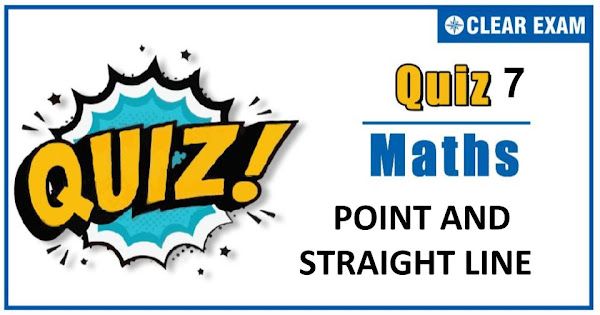## [LATEST]\$type=sticky\$show=home\$rm=0\$va=0\$count=4\$va=0

Dear Readers, As per analysis for previous years, it has been observed that students preparing for JEE MAINS find Mathematics out of all the sections to be complex to handle and the majority of them are not able to comprehend the reason behind it. This problem arises especially because these aspirants appearing for the examination are more inclined to have a keen interest in Mathematics due to their ENGINEERING background.
Furthermore, sections such as Mathematics are dominantly based on theories, laws, numerical in comparison to a section of Engineering which is more of fact-based, Physics, and includes substantial explanations. By using the table given below, you easily and directly access to the topics and respective links of MCQs. Moreover, to make learning smooth and efficient, all the questions come with their supportive solutions to make utilization of time even more productive. Students will be covered for all their studies as the topics are available from basics to even the most advanced.
.

Q1. The angle between the pair of lines (x2+y2)sin2α=(x cos⁡θ-ysin⁡θ)2 is
•  θ
•
•  α
•
Solution
d)
Q2.Distance between the two parallel lines y=2x+7 and y=2x+5 is
•  √5/2
•  2/5
•  2/√5
•  1/√5
Solution
c) On comparing the given lines with
y=m1x+c1 and y=m2x+c2,we get
m1=2 and c1=7
and m2=2 and c2=5
∴Required distance=|c1-c2|/√(m2+1)
=(|7-5|)/√((2)2+1)=2/√5
Q3.  The distance between the pair of parallel lines x2+2xy+y2-8ax-8ay-9a2=0 is
•  x+y=ab
•  x+y=a+b
•  ax+by=a2
•  bx+ay=b2
Solution

d) Here a=1,h=1,f=-4a,g=-4a,c=-9a
Now, required distance
=|2√((f2-bc)/b(b+a) )|
=|2√((16a2+9a2)/1(1+1) )|
=|2√((25a2)/2)|=5a/√2∙2
=5√2 a

Q4. The straight line 3x+y=9 divided the line segment joining the points (1, 3) and (2,7) in the ratio
•  3:4 externally
•  3:4 internally
•  4:5 internally
•  5:6 externally
Solution
b) Required ratio is given by -(3×1+3-9)/(3×2+7-9 ) =3/4 ie,3:4 internally
Q5.The line x/a - y/b=1 cuts the x-axis at P. The equation of the line through P perpendicular to the given line is
•  x+y=ab
•  x+y=a+b
•  ax+by=a2
•  bx+ay=b2
Solution
c) Line perpendicular to the given line x/a-y/b=1 is 1/b x+1/a y+λ=0 …(i) According to the question, line (i) is Passing through the point P(a,0) a/b+0+λ=0 ⇒ λ=-a/b
Q6. The equation of the image of the lines y=|x| in the line mirror x=2 is
•  y=|x-4|
•  |y|=x+4
•  |y|+4=x
•  None of these
Solution
a) The required lines are obtaining by shifting the origin at (4,0). So, the required equation is y=|x-4|
Q7.A line passing through origin and is perpendicular to two given lines 2x+y+6=0 and 4x+2y-9=0. The ratio
in which the origin divides this line, is
•  1:2
•   2:1
•  4:2
•  4:3
Solution

d) Equation of line perpendicular to 2x+y+6=0 and passes through origin is x-2y=0
Now,point of intersection of 2x+y+6=0 and x-2y=0 is(-12/5,-6/5)
Similarly,point of intersection of x-2y=0 and 4x+2y-9=0 is (9/5,9/10)
Let the origin divide the line x-2y=0 in the ratio λ:1
∴ x=(9/5 λ-12/5)/(λ+1)=0 ⇒ 9/5 λ=12/5
⇒ λ=12/9=4/3
Q8.The centroid of the triangle formed by the pair of straight lines 12x2-20xy+7y2=0 and the line 2x-3y+4=0 is
•  (-7/3,7/3)
•  (-8/3,8/3)
•  (8/3,8/3)
•  (4/3,4/3)
Solution

(c) The separate equation of pair of straight lines of 12x2-20xy+7y2=0 are 6x-7y=0 and 2x-y=0
Thus, equation of sides of triangle are
6x-7y=0 …(i)
2x-y=0 …(ii)
and 2x-3y+4=0 …(iii)
On solving these equations, we get the vertices of a triangle A(0,0);B(1,2)and C(7,6)
∴ Centroid of triangle is
((0+1+7)/3,(0+2+6)/3)=(8/3,8/3)
Q9.  If a≠b≠c, then the equations
(b-c)x+(c-a)y+(a-b)=0
and,(b3-c3)x+(c3-a3)y+(a3-b3)=0
will represent the same line, if
•  a+b=-c
•  c+a=-b
•  b+c=-a
•  a+b+c=0
Solution
d) The equations will represent the same line if
(b3-c3)/(b-c)=(c3-a3)/(c-a)=(a3-b3)/(a-b)
⇒b2+bc+c2=c2+ca+a2=a2+ab+b2
⇒b2+bc+c2=c2+ca+a2 and b2+bc+c2=a2+ab+b2
⇒b2-a2+bc-ca=0 and c2-a2+bc-ab=0
⇒(b-a)(b+a+c)=0 and (c-a)(c+a+b)=0
⇒a+b+c=0
Q10. If a line with y-intercept 2, is perpendicular to the line 3x-2y=6, then its x- intercept is
•  1
•   2
•   -4
•  3
Solution
(d) Let the equation of perpendicular line to the line
3x-2y=6 is 3y+2x=c …(i)
Since, it passes through (0, 2)
∴c=6
On putting the value of c in Eq. (i) we get 3y+2x=6
⇒x/3+y/2=1
Hence, x-intercept is 3.#### Written by: AUTHORNAME

AUTHORDESCRIPTION## Want to know more

Please fill in the details below:

## Latest NEET Articles\$type=three\$c=3\$author=hide\$comment=hide\$rm=hide\$date=hide\$snippet=hide

Name

ltr
item
BEST NEET COACHING CENTER | BEST IIT JEE COACHING INSTITUTE | BEST NEET & IIT JEE COACHING: Point and Straight Line Quiz-7
Point and Straight Line Quiz-7
https://1.bp.blogspot.com/-Q3Hd0s2Q5AA/X6UsoJZPLvI/AAAAAAAAKaU/SzzpsW9lpfMOAbDr5qmEODcFNHra3wr_wCLcBGAsYHQ/s600/Quiz%2BImage%2B20.jpg
https://1.bp.blogspot.com/-Q3Hd0s2Q5AA/X6UsoJZPLvI/AAAAAAAAKaU/SzzpsW9lpfMOAbDr5qmEODcFNHra3wr_wCLcBGAsYHQ/s72-c/Quiz%2BImage%2B20.jpg
BEST NEET COACHING CENTER | BEST IIT JEE COACHING INSTITUTE | BEST NEET & IIT JEE COACHING
https://www.cleariitmedical.com/2020/11/point-and-straight-line-quiz-7.html
https://www.cleariitmedical.com/
https://www.cleariitmedical.com/
https://www.cleariitmedical.com/2020/11/point-and-straight-line-quiz-7.html
true
7783647550433378923
UTF-8

STAY CONNECTED